Ex 10.4

Chapter 10 Class 11 Conic Sections
Serial order wise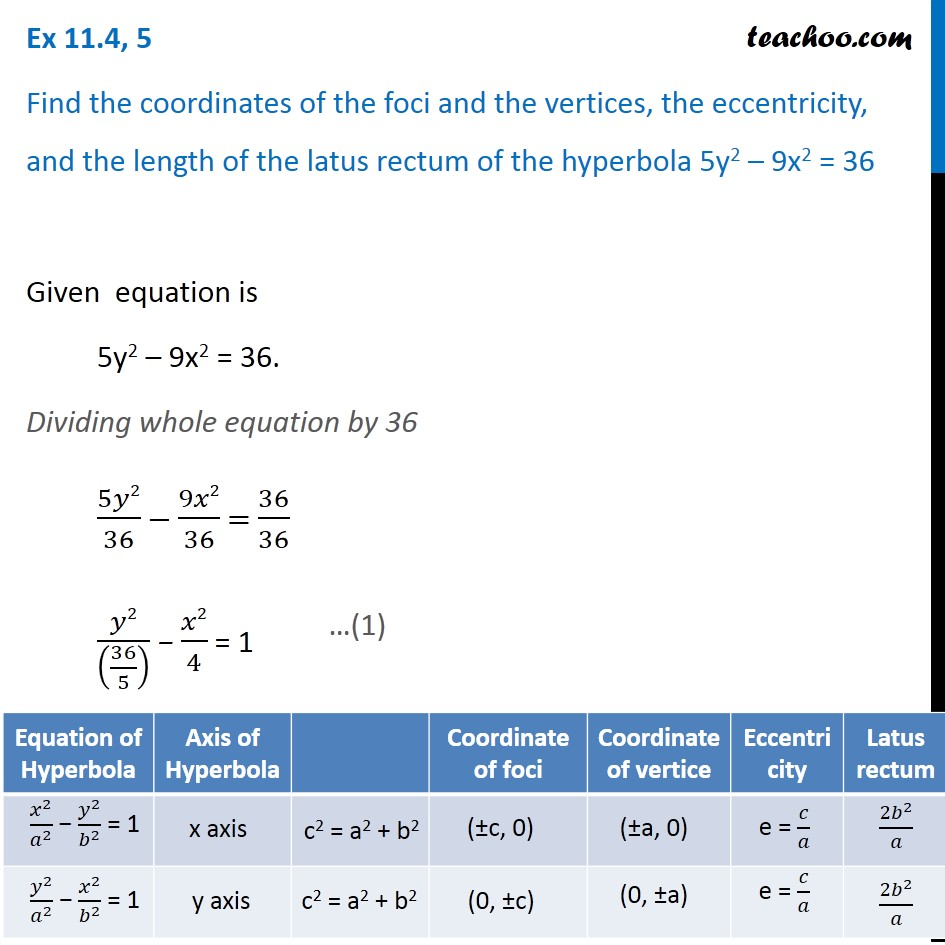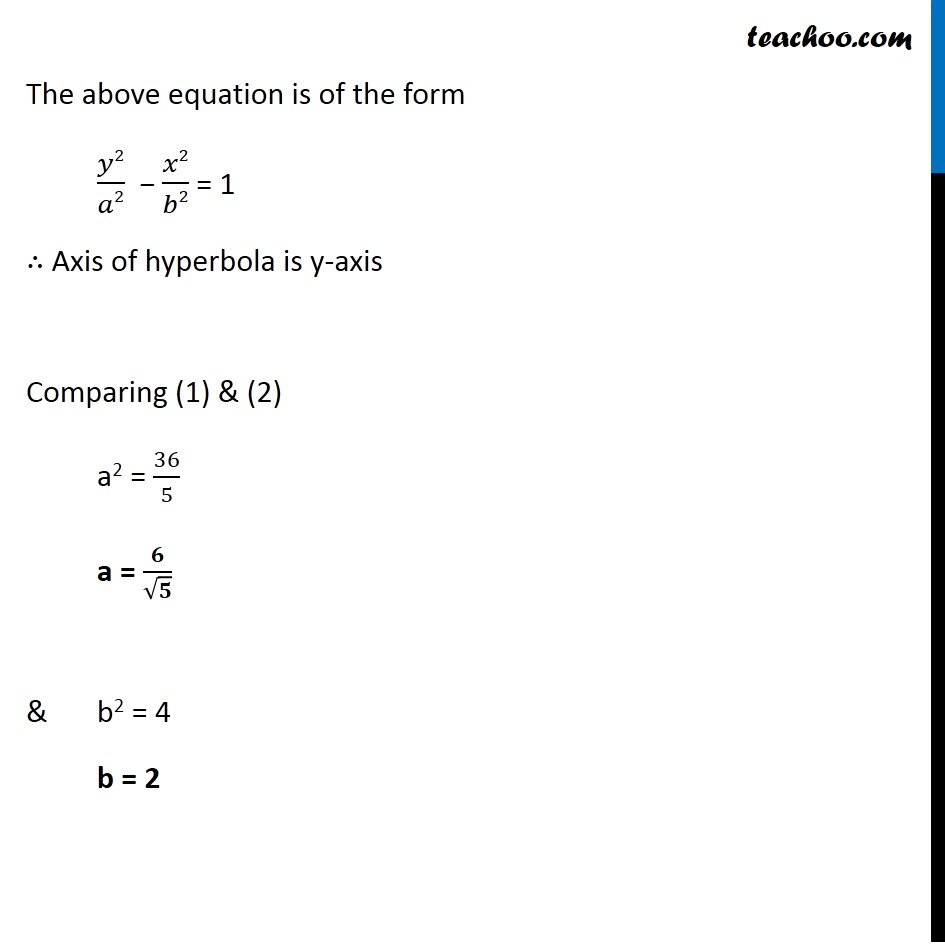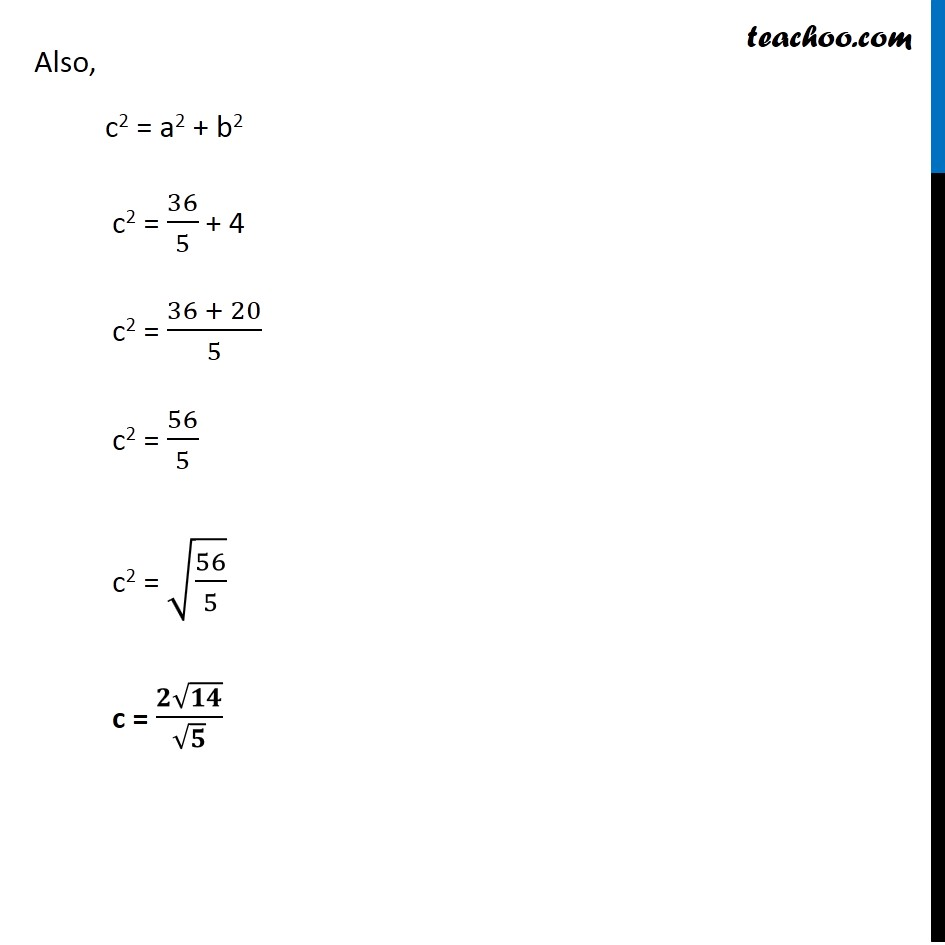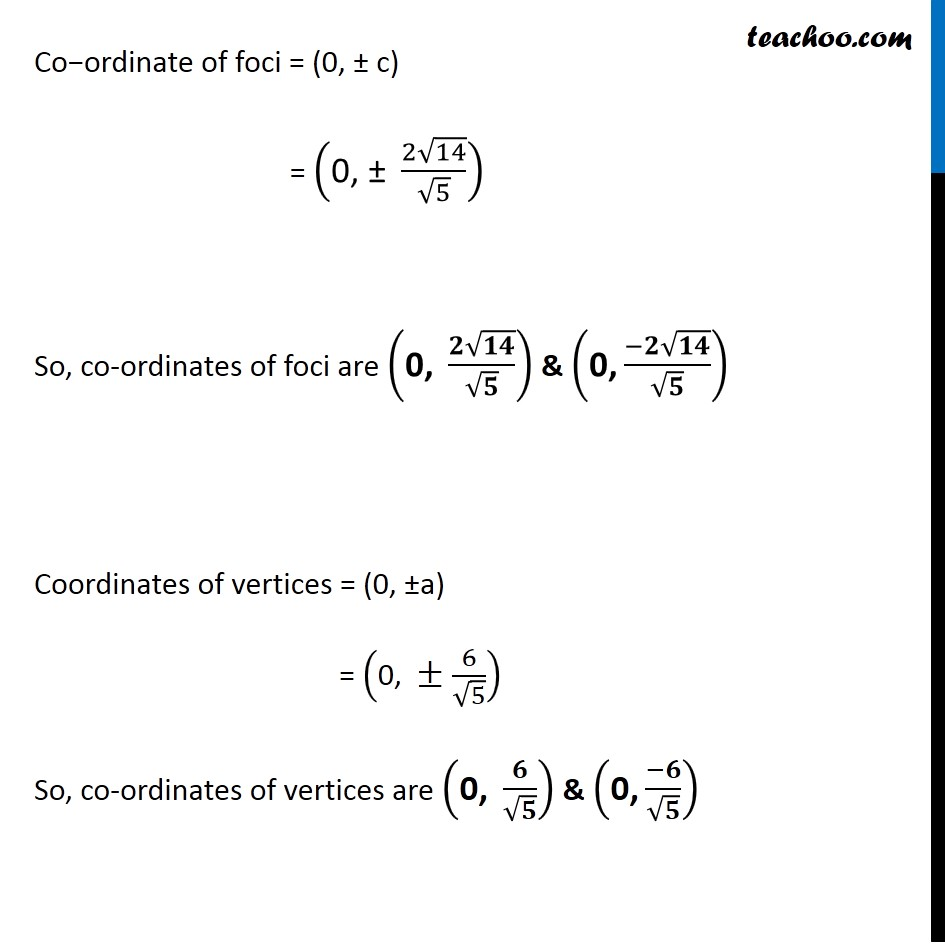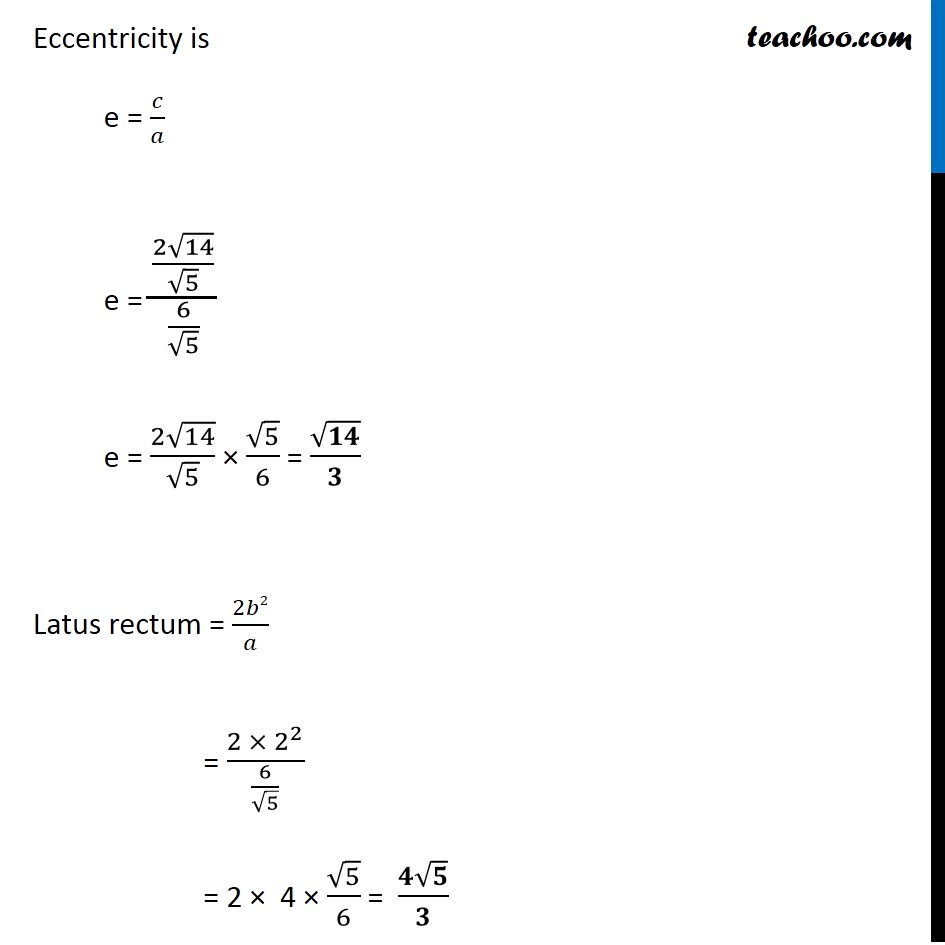Learn in your speed, with individual attention - Teachoo Maths 1-on-1 Class

### Transcript

Ex 10.4, 5 Find the coordinates of the foci and the vertices, the eccentricity, and the length of the latus rectum of the hyperbola 5y2 – 9x2 = 36 Given equation is 5y2 – 9x2 = 36. Dividing whole equation by 36 5𝑦2/36 − 9𝑥2/36 = 36/36 𝑦2/((36/5) ) − 𝑥2/4 = 1 The above equation is of the form 𝑦2/𝑎2 − 𝑥2/𝑏2 = 1 ∴ Axis of hyperbola is y-axis Comparing (1) & (2) a2 = 36/5 a = 𝟔/√𝟓 & b2 = 4 b = 2 Also, c2 = a2 + b2 c2 = 36/5 + 4 c2 = (36 + 20)/5 c2 = 56/5 c2 = √(56/5) c = (𝟐√𝟏𝟒)/√𝟓 Co−ordinate of foci = (0, ± c) = ("0, ± " (2√14)/√5) So, co-ordinates of foci are ("0, " (𝟐√𝟏𝟒)/√𝟓) & ("0," (−𝟐√𝟏𝟒)/√𝟓) Coordinates of vertices = (0, ±a) = ("0, " ±6/√5) So, co-ordinates of vertices are ("0, " 𝟔/√𝟓) & ("0," (−𝟔)/√𝟓) Eccentricity is e = 𝑐/𝑎 e = ((2√14)/√5)/(6/√5) e = (2√14)/√5 × √5/6 = √𝟏𝟒/𝟑 Latus rectum = 2𝑏2/𝑎 = (2 × 2^2)/(6/√5) = 2 × 4 × √5/6 = (𝟒√𝟓)/𝟑